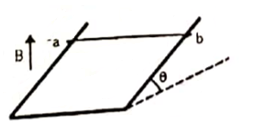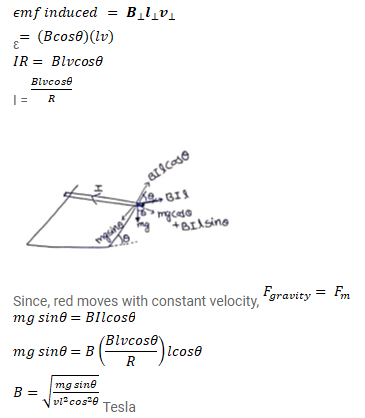# A wire ab of length I, mass m and resistance R slides on a smooth,

Question:

A wire ab of length I, mass $m$ and resistance $R$ slides on a smooth, thick pair of metallic rails joined at the bottom as shown in figure. The plane of the rails makes an angle $\theta$ with the horizontal. A vertical magnetic field B exists in the region. If the wire slides on the rails at a constant speed v, show that

$B=\sqrt{\frac{m g \sin \theta}{v l^{2} \cos ^{2} \theta}}$Solution: